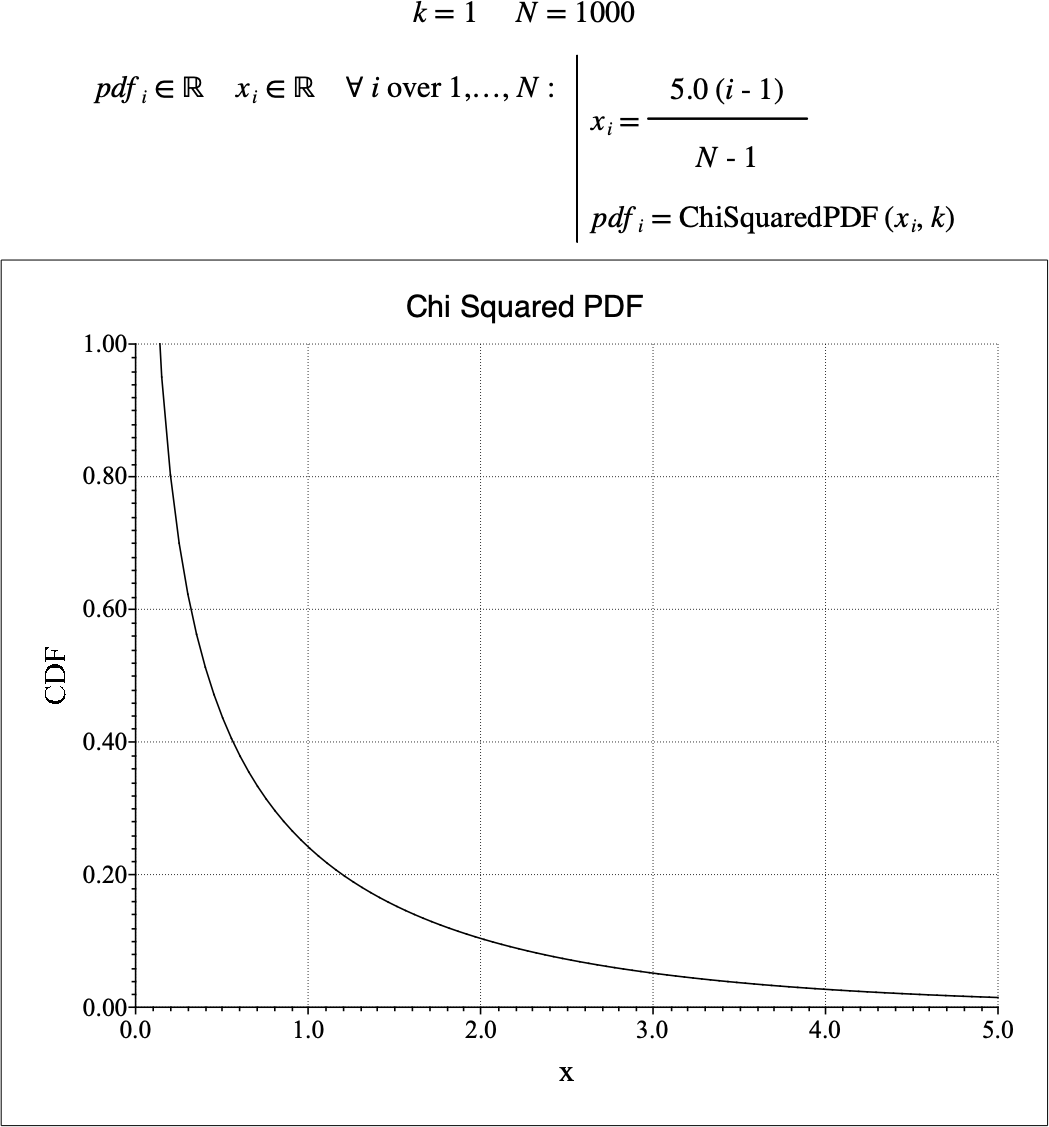# $$\text{ChiSquaredPDF}$$¶

You can use the $$\text{ChiSquaredPDF}$$ function to calculate the probability density function (PDF) of the chi-squared distribution.

You can use the \chisquaredp backslash command to insert this function.

The following variants of this function are available:

• $$\text{real } \text{ChiSquaredPDF} \left ( \text{<x>}, \text{<k>} \right )$$

Where $$x$$ and $$k$$ are scalar values indicating the value of interest and the degrees of freedom respectively. The function is defined for all $$k \in \mathbb{Z}$$ where $$k > 0$$ and where $$0 < x$$ if $$k = 1$$ and $$0 \leq x$$ for all other values of $$k$$.

The value is calculated directly using the relation:

$\text{ChiSquaredPDF} \left ( x, k \right ) = \frac{1} {2 ^ {\frac{k}{2}} \Gamma \left ( \frac{k}{2} \right )} x ^ { \frac{k}{2} - 1 } e ^ {- \frac{x}{2}}$

The chi-squared distribution represents the sum of the squares of $$k$$ standard normally distributed random variates.

Figure 117 shows the basic use of the $$\text{ChiSquaredPDF}$$ function.Figure 117 Example Use Of the ChiSquaredPDF Function